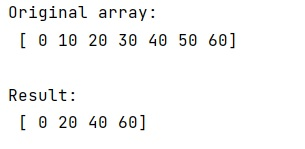# Select all elements in a NumPy array except for a sequence of indices?

Learn, how can we select all elements in a NumPy array except for a sequence of indices in Python?
Submitted by Pranit Sharma, on March 27, 2023

## Problem statement

Suppose that we are given a numpy array and a list of indices. We need to select every element of this array except those which are at the position corresponding to the index's elements.

For example, if we have an array [1,2,3,4] and a list of indices as [2,3] then we will select [1,2] from the array but not [2,3] which are at the position of [2,3] (elements of indices array too).

## Select all elements except for a sequence of indices

To select all elements except for a sequence of indices, we use the numpy.delete() function. The advantage of this function is that it does not modify the array. We will pass the list of indices and input array in this function and it will remove all the elements at the position of the index's elements.

Let us understand with the help of an example,

## Python program to select all elements in a NumPy array except for a sequence of indices

```# Import numpy
import numpy as np

# Creating a numpy array
arr = np.array([0,10,20,30,40,50,60])

# Display original array
print("Original array:\n",arr,"\n")

# List of indices
ind = [1,3,5]

# Selecting all elements except list of indices
res = np.delete(arr, ind)

# Display result
print("Result:\n",res)
```

## Output